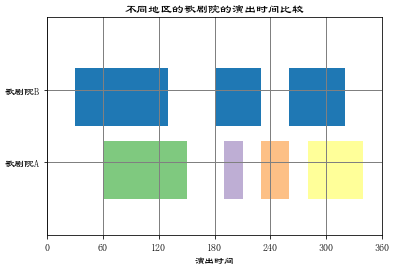# python可视化---间断条形图broken_barh()

2019/03/01 20:47

import matplotlib.pyplot as plt
import matplotlib as mpl
import numpy as np

mpl.rcParams["font.sans-serif"] = ["LiSu"]
mpl.rcParams["axes.unicode_minus"] = False

plt.broken_barh([(30, 100), (180, 50), (260, 60)], (20, 8), facecolors="#1f78b4")
plt.broken_barh([(60, 90), (190, 20), (230, 30), (280, 60)], (10, 8), facecolors=(
"#7fc97f", "#beaed4", "#fdc086", "#ffff99"))

plt.xlim(0, 360)
plt.ylim(5, 35)
plt.xlabel("演出时间")

plt.xticks(np.arange(0, 361, 60))
plt.yticks([15, 25], ["歌剧院A", "歌剧院B"])

plt.grid(ls="-", lw=1, color="gray")

plt.title("不同地区的歌剧院的演出时间比较")

plt.show()0
0 收藏

### 作者的其它热门文章0 评论
0 收藏
0